# Scilab Examples – 2D plots

This is a practical approach to plots in Scilab. Several examples are shown to explore the capabilities of this software. After each line of code is explained, we show the result produced.

### Simplest forms

Function plot2d plots a set of 2D curves. If you are familiar with the Matlab plot syntax, you could use function plot. If x and y are vectors, plot2d(x, y, <opt_args>) plots vector y versus vector x. x and y vectors must have the same size.

x = 0 : .1 : 10*%pi;
y = x .* cos(x);
// Plot as used in Matlab
plot(x, y)
// Add title, labels and legend
title('x * cos(x)')
xlabel('x'); ylabel('y');
legend('y = x * cos(x)');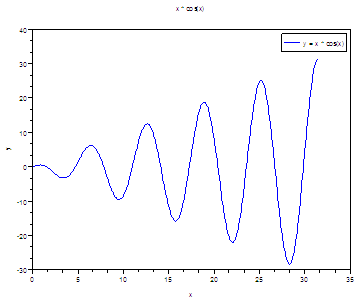// Multiple plot with captions and given tics + style
x = [0 : 0.1 : 2*%pi]';
plot2d(x, [sin(x) sin(2*x)], [2, 5],...
leg = "sin(x)@sin(2x)", nax = [2, 5, 1, 5],...
rect = [0, -1, 2*%pi, 1])

// [2, 5] means lines in blue (2) and red (5)
// leg = "sin(x)@sin(2x)" are the legends of the lines
// nax = [2, 5, 1, 5] are the tics and subtics of the
// horizontal and
// vertical axis
// rect = [0, -1, 2*%pi, 1] are the bounds of the axis,
// from (0, -1) to (2pi, 1).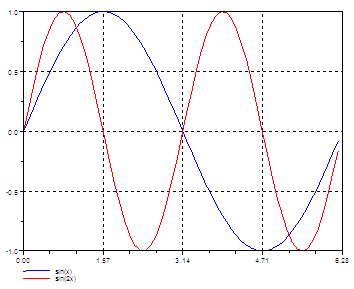### Logarithmic Axis – Plots

t = linspace(0, 2*%pi, 300);
x = exp(-t);
y = t;
// Use the logflag option to define logarithmic or normal axis
// n for normal, l for logarithmic.
plot2d(x, y, logflag = "ln");
xgrid;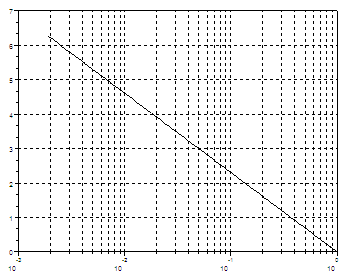// Open a new figure window with scf
scf(); plot2d(t, exp(t), logflag = "nl"); xgrid;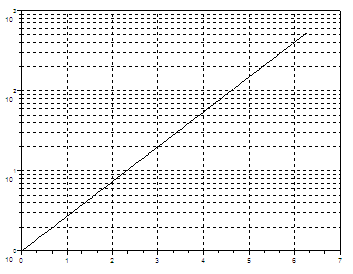// Both axis are logarithmic now
scf(); plot2d(exp(t), 50 + exp(2*t), logflag = "ll") ; xgrid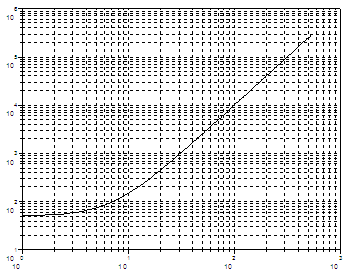### Polar Plots

polarplot - Plot polar coordinates
polarplot(theta, rho, <opt_args>)

t = linspace(0, 2*%pi, 100);
r = sqrt(abs(2 * cos(5*t)));
// Plot in polar coordinates
polarplot(t, r);Polar Plots in Matlab

### Histograms in Scilab

histplot - plot a histogram
histplot(bins, data, <opt_args>)

// Define your data or get it somehow
y = 10 * rand(500, 1);
// Create the histogram. The 5 at the beginning means 5 bins.
// The 2 at the end means lines in blue.
histplot(5, y, 2)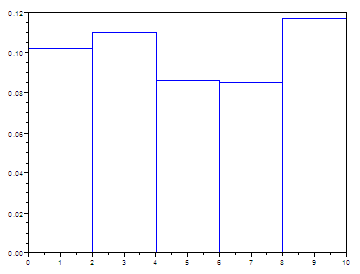Histograms in Matlab

### Pie Plots

pie - draw a pie
pie(x [, sp [,txt]])

x is a scalar or a vector of real, positive numbers.
sp is a real scalar or vector.
txt means a cell or a vector of strings.

pie([1 3 4 5], [1 0 1 0],...
["Part1 Exp.","Part2","Part3 Exp.", "P4"]);

// [1 3 4 5] is the number and size of sectors
// [1 0 1 0], 1 for exploded sector, 0 for nonexploded
// ["Part1 Exp.","Part2","Part3 Exp.", "P4"] is the associated
// text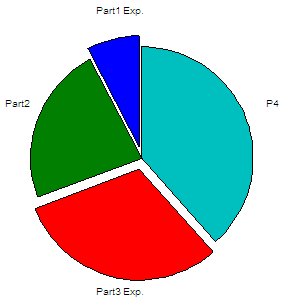Pie Plots in Matlab

### Stems – Vertical lines

plot2d3 - 2D plot (vertical bars)
plot2d3([logflags,] x,y,[style,strf,leg,rect,nax])
plot2d3(x,y <,opt_args>)

plot2d3 is the same as plot2d but curves are plotted using vertical bars. By default, successive plots are superposed. To clear the previous plot, use clf().

x = [0 : 0.2 : 4*%pi];
// Define your functions and plot.
// The default color is black
plot2d3(x, sin(x))
// 5 at the end means red line
plot2d3(x, sin(2*x), 5)
// Superimpose a red envelope on the red lines
plot2d(x, sin(2*x), 5)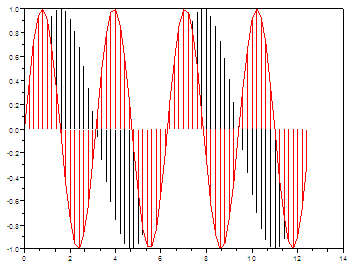Stems in Matlab

### Stairs Function

Use plot2d2 for this purpose.

// Define your variables and functions
t = linspace(-2*%pi, 2*%pi, 200);
r = sqrt( abs(2 * cos(4*t)) );
y = r .* cos(t);
// Plot using a red line (5) and
// bound axis from (-1.5, 0) to (1.6, 1.5)
plot2d2(t, y, 5, rect = [-1.5, 0, 1.6, 1.5]);
// Add title, labels, legend and grid
title('Stairs/Steps Function');
xlabel('x'); ylabel('y');
legend('y = Step function');
xgrid;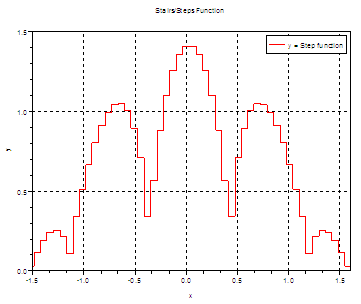From 'Scilab Examples' to Matlab home

From 'Scilab Examples' to Scilab Menu